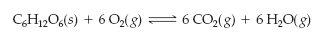×
Get Full Access to Introductory Chemistry - 5 Edition - Chapter 15 - Problem 73p
Get Full Access to Introductory Chemistry - 5 Edition - Chapter 15 - Problem 73p

×

# Solved: This reaction is exothermic. Predict the effect (shift right, shift left, or noISBN: 9780321910295 34

## Solution for problem 73P Chapter 15

Introductory Chemistry | 5th Edition

• Textbook Solutions
• 2901 Step-by-step solutions solved by professors and subject experts
• Get 24/7 help from StudySoup virtual teaching assistantsIntroductory Chemistry | 5th Edition

4 5 1 417 Reviews
15
4
Problem 73P

This reaction is exothermic.Predict the effect (shift right, shift left, or no effect) of these changes.

(a) increasing the reaction temperature

(b) decreasing the reaction temperature

Step-by-Step Solution:
Step 1 of 3

Solution: Here, we are going to predict the effect of the changes in the given reaction.

Step1:

Le Chatelier’s principle states that, a change in any of the factors that determine the equilibrium conditions of a system will cause the system to change in such a manner so as to reduce or to counteract the effect of the change.

For an exothermic reaction, increase in temperature decreases the value of the equilibrium constant and for an endothermic reaction, the equilibrium constant increases as the temperature increases.

Step2:

Since, the given reaction is exothermic, therefore,

1. Increasing the reaction temperature will shift the equilibrium to the left.
2. Decreasing the reaction temperature will shift the equilibrium to the right.

---------------------------------

Step 2 of 3

Step 3 of 3

##### ISBN: 9780321910295

Introductory Chemistry was written by and is associated to the ISBN: 9780321910295. Since the solution to 73P from 15 chapter was answered, more than 278 students have viewed the full step-by-step answer. The answer to “?This reaction is exothermic. Predict the effect (shift right, shift left, or no effect) of these changes.(a) increasing the reaction temperature(b) decreasing the reaction temperature” is broken down into a number of easy to follow steps, and 25 words. This full solution covers the following key subjects: reaction, shift, temperature, effect, predict. This expansive textbook survival guide covers 19 chapters, and 2046 solutions. The full step-by-step solution to problem: 73P from chapter: 15 was answered by , our top Chemistry solution expert on 05/06/17, 06:45PM. This textbook survival guide was created for the textbook: Introductory Chemistry, edition: 5.

Unlock Textbook Solution